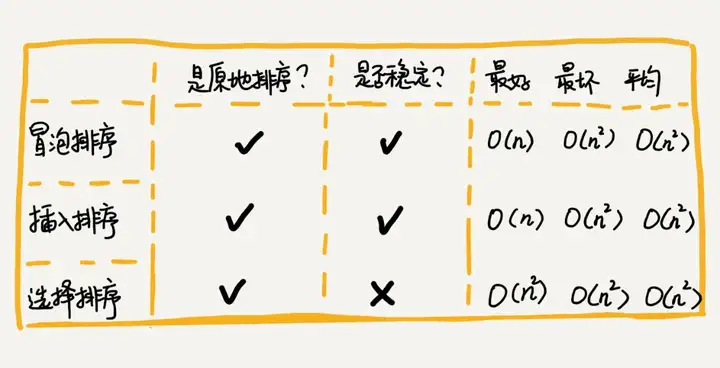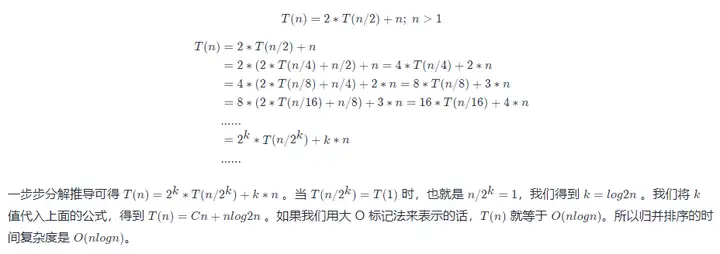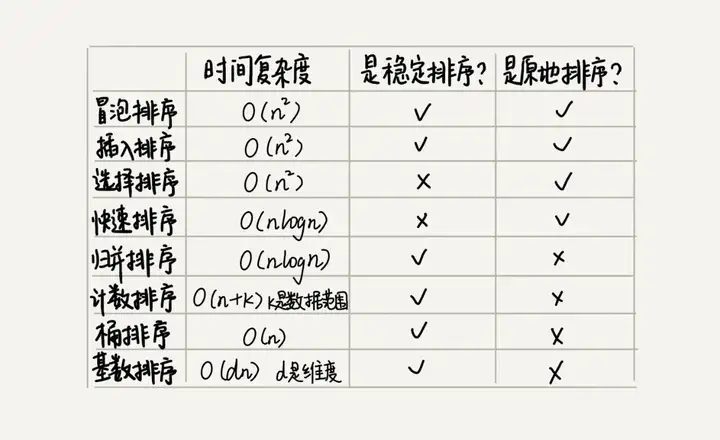# 簡述幾種常用的排序演算法

## 一，氣泡排序（Bubble Sort）

``````// 將資料從小到大排序
void bubbleSort(int array[], int n){
if (n<=1) return;
for(int i=0; i<n; i++){
for(int j=0; j<n-i; j++){
if (temp > a[j+1]){
temp = array[j]
a[j] = a[j+1];
a[j+1] = temp;
}
}
}
}``````

``````// 將資料從小到大排序
void bubbleSort(int array[], int n){
if (n<=1) return;
for(int i=0; i<n; i++){
// 提前退出冒泡迴圈發標志位
bool flag = False;
for(int j=0; j<n-i; j++){
if (temp > a[j+1]){
temp = array[j]
a[j] = a[j+1];
a[j+1] = temp;
flag = True; // 表示本次冒泡操作存在資料交換
}
}
if(!flag) break; // 沒有資料交換，提交退出
}
}``````

1. 冒泡過程只涉及相鄰元素的交換，只需要常量級的臨時空間，故空間複雜度為 O(1)O(1)，是原地排序演算法
2. 當有相鄰的兩個元素大小相等的時候，我們不做交換，相同大小的資料在排序前後不會改變順序，所以是穩定排序演算法
3. 最壞情況和平均時間複雜度都為 O(n2)O(n2)，最好時間複雜度是 O(n)O(n)。

## 二，插入排序（Insertion Sort）

1. 插入排序演算法將陣列中的資料分為兩個區間：已排序區間和未排序區間。最初始的已排序區間只有一個元素，就是陣列的第一個元素。
2. 插入排序演算法的核心思想就是取未排序區間的一個元素，在已排序區間中找到一個合適的位置插入，並保證已排序區間資料一直有序。
3. 重複這個過程，直到未排序區間元素為空，則演算法結束。

``````void InsertSort(int a[], int n){
if (n <= 1) return;
for (int i = 1; i < n; i++) // 未排序區間範圍
{
key  = a[i]; // 待排序第一個元素
int j = i - 1; // 已排序區間末尾元素
// 從尾到頭查詢插入點方法
while(key < a[j] && j >= 0){ // 元素比較
a[j+1] = a[j]; // 資料向後移動一位
j--;
}
a[j+1] = key; // 插入資料
}
}``````

1. 插入排序並不需要額外儲存空間，空間複雜度是 O(1)O(1)，所以插入排序也是一個原地排序演算法。
2. 在插入排序中，對於值相同的元素，我們可以選擇將後面出現的元素，插入到前面出現元素的後面，這樣就可以保持原有的前後順序不變，所以插入排序是穩定的排序演算法。
3. 最壞情況和平均時間複雜度都為 O(n2)O(n2)，最好時間複雜度是 O(n)O(n)。

## 三，選擇排序（Selection Sort）

``````void SelectSort(int a[], int n){
for(int i=0; i<n; i++){
int minIndex = i;
for(int j = i;j<n;j++){
if (a[j] < a[minIndex]) minIndex = j;
}
if (minIndex != i){
temp = a[i];
a[i] = a[minIndex];
a[minIndex] = temp;
}
}
}``````

### 冒泡插入選擇排序總結## 四，歸併排序（Merge Sort）

``````遞推公式：
merge_sort(p…r) = merge(merge_sort(p…q), merge_sort(q+1…r))

p >= r 不用再繼續分解，即區間陣列元素為 1 ``````

``````merge_sort(A, n){
merge_sort_c(A, 0, n-1)
}
merge_sort_c(A, p, r){
// 遞迴終止條件
if (p>=r) then return
// 取 p、r 中間的位置為 q
q = (p+r)/2
// 分治遞迴
merge_sort_c(A[p, q], p, q)
merge_sort_c(A[q+1, r], q+1, r)
// 將A[p...q]和A[q+1...r]合併為A[p...r]
merge(A[p...r], A[p...q], A[q+1...r])
}``````

### 4.1，歸併排序效能分析

1，歸併排序是一個穩定的排序演算法。分析：虛擬碼中 merge_sort_c() 函式只是分解問題並沒有涉及移動元素和比較大小，真正的元素比較和資料移動在 merge() 函式部分。在合併過程中保證值相同的元素合併前後的順序不變，歸併排序排序就是一個穩定的排序演算法。

2，歸併排序的執行效率與要排序的原始陣列的有序程度無關，所以其時間複雜度是非常穩定的，不管是最好情況、最壞情況，還是平均情況，時間複雜度都是 O(nlogn)O(nlogn)。分析：不僅遞迴求解的問題可以寫成遞推公式，遞迴程式碼的時間複雜度也可以寫成遞推公式：3，空間複雜度是 O(n)。分析：遞迴程式碼的空間複雜度並不能像時間複雜度那樣累加。儘管演算法的每次合併操作都需要申請額外的記憶體空間，但在合併完成之後，臨時開闢的記憶體空間就被釋放掉了。在任意時刻，CPU 只會有一個函式在執行，也就只會有一個臨時的記憶體空間在使用。臨時記憶體空間最大也不會超過 n 個數據的大小，所以空間複雜度是 O(n)O(n)。

## 五，快速排序（Quicksort）

``````遞推公式：
quick_sort(p,r) = quick_sort(p, q-1) + quick_sort(q, r)

p >= r``````

### 歸併排序和快速排序總結## 參考資料

• 排序（上）：為什麼插入排序比氣泡排序更受歡迎？
• 排序（下）：如何用快排思想在O(n)內查詢第K大元素？

「其他文章」